• 1. 与简单线性回归区别(simple linear ...2. 多元回归模型 y=β0＋β１x1+β2x2+ … +βpxp+ε 其中：β0，β１，β2… βp是参数 ε是误差值 3. 多元回归方程 E(y)=β0＋β１x1+β2x2+ … +βpxp 4. 估计
1. 与简单线性回归区别(simple linear regression)
多个自变量(x)

2. 多元回归模型
y=β0＋β１x1+β2x2+ … +βpxp+ε
其中：β0，β１，β2… βp是参数
ε是误差值

3. 多元回归方程
E(y)=β0＋β１x1+β2x2+ … +βpxp

4. 估计多元回归方程:
y_hat=b0＋b１x1+b2x2+ … +bpxp

一个样本被用来计算β0，β１，β2… βp的点估计b0, b1, b2,…, bp

5. 估计流程  (与简单线性回归类似）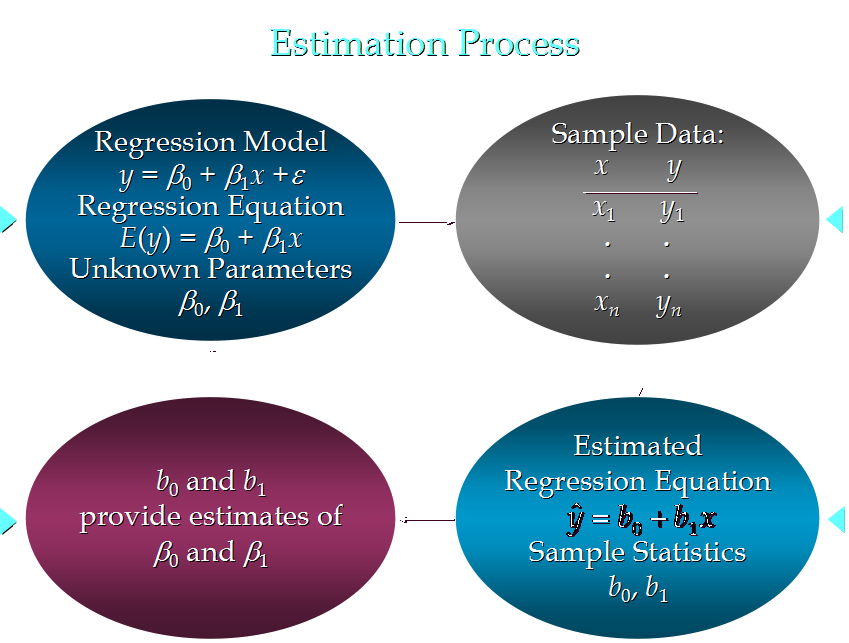6. 估计方法
使sum of squares最小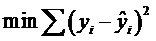运算与简单线性回归类似，涉及到线性代数和矩阵代数的运算 7. 例子

一家快递公司送货：X1： 运输里程 X2： 运输次数   Y：总运输时间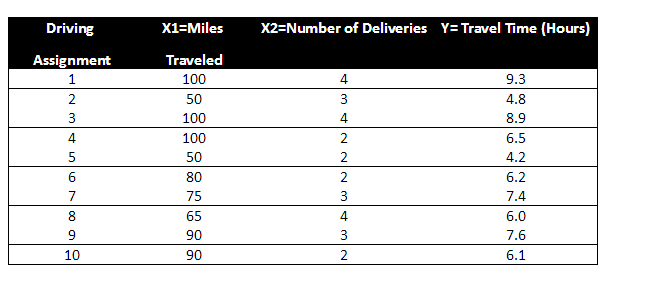目的，求出b0, b1,…. bp：
y_hat=b0＋b１x1+b2x2+ … +bpxp

Time = b0+ b1*Miles + b2 * Deliveries
Time = -0.869 + 0.0611 Miles + 0.923 Deliveries

8. 描述参数含义
b0: 平均每多运送一英里，运输时间延长0.0611 小时
b1: 平均每多一次运输，运输时间延长 0.923 小时

9. 预测
如果一个运输任务是跑102英里，运输6次，预计多少小时？
Time = -0.869 +0.0611 102+ 0.923  6= 10.9 (小时）

10. 如果自变量中有分类型变量(categorical data) , 如何处理？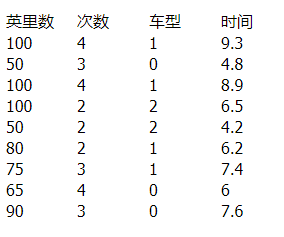11. 关于误差的分布
误差ε是一个随机变量，均值为0
ε的方差对于所有的自变量来说相等
所有ε的值是独立的
ε满足正态分布，并且通过β0＋β１x1+β2x2+ … +βpxp反映y的期望值

# -*- coding:utf-8 -*-
from numpy import genfromtxt
from sklearn import linear_model
# genfromtxt函数
#  genfromtxt函数创建数组表格数据
#  genfromtxt主要执行两个循环运算。第一个循环将文件的每一行转换成字符串序列。第二个循环将每个字符串序列转换为相应的数据类型。
#  详细用法参考：https://docs.scipy.org/doc/numpy/reference/generated/numpy.genfromtxt.html
#  附加numpy.savetxt该函数能够将数据存储为 CSV 格式：https://docs.scipy.org/doc/numpy/reference/generated/numpy.savetxt.html

datapath=r"Delivery_Dummy.csv"
data = genfromtxt(datapath,delimiter=",")
#delimiter: the str used to separate data. 横纵坐标以 ',' 分割，因此给 delimiter 传入 ','。delimiter是区分横纵坐标的
# skip_header: the number of lines to skip at the beginning of the file. 这个参数是跳过表头信息的

x = data[1:,:-1]
y = data[1:,-1]
print x
print y

mlr = linear_model.LinearRegression()

mlr.fit(x, y)

print mlr
print "coef:"
print mlr.coef_
print "intercept"
print mlr.intercept_

xPredict =  [90,2,0,0,1]
yPredict = mlr.predict(xPredict)

print "predict:"
print yPredict
展开全文regression Python sklearn
• ## 多元线性回归模型

万次阅读 多人点赞 2019-07-02 19:22:12
多元线性回归模型通常用来研究一个应变量依赖多个自变量的变化关系，如果二者的以来关系可以用线性形式来刻画，则可以建立多元线性模型来进行分析。 1.模型简介 1.1模型的结构 多元线性回归模型通常用来描述变脸y和x...
多元线性回归模型通常用来研究一个应变量依赖多个自变量的变化关系，如果二者的以来关系可以用线性形式来刻画，则可以建立多元线性模型来进行分析。
1.模型简介
1.1模型的结构
多元线性回归模型通常用来描述变量y和x之间的随机线性关系，即：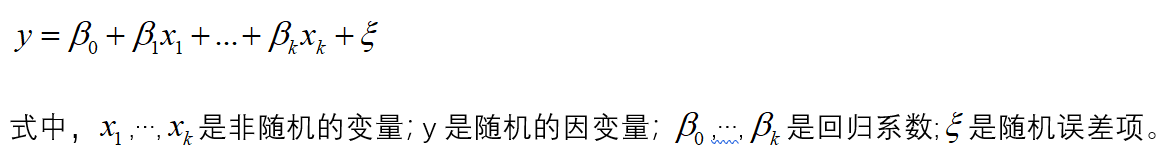如果对y和x进行了x次观测，得到n组观察值yi,x1i,…,xki(i=1,2,…,n),他们满足一下关系式：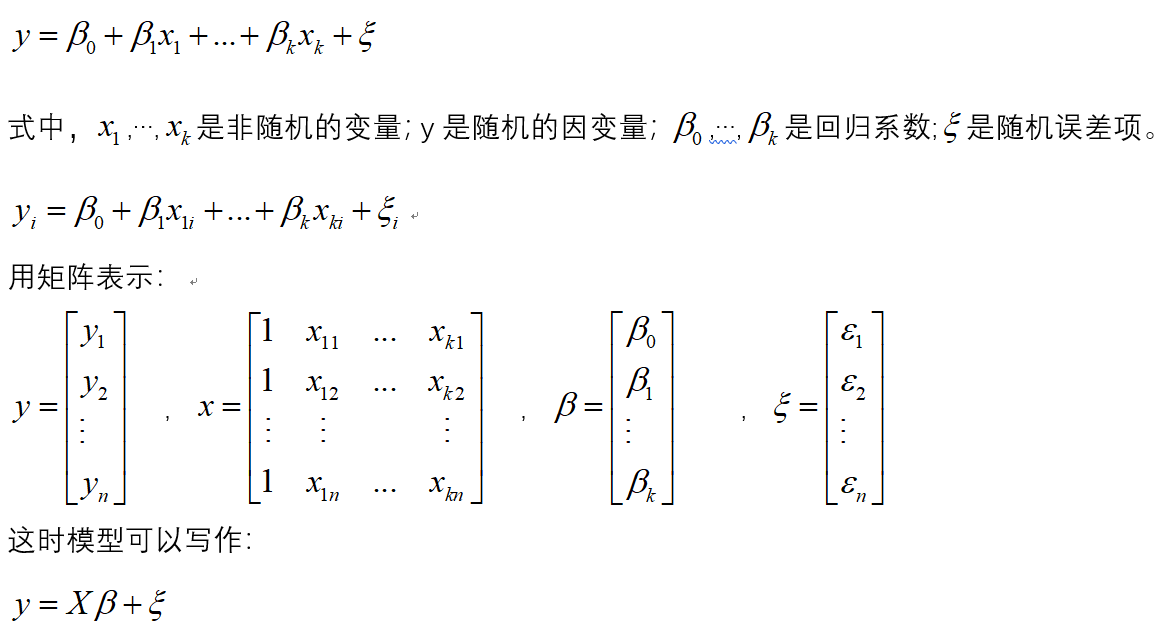1.2模型参数的检验
在正态假定下，如果X是列满秩的，则普通线性回归模型的参数最小二乘估计为：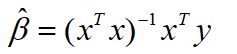于是y的估计值为：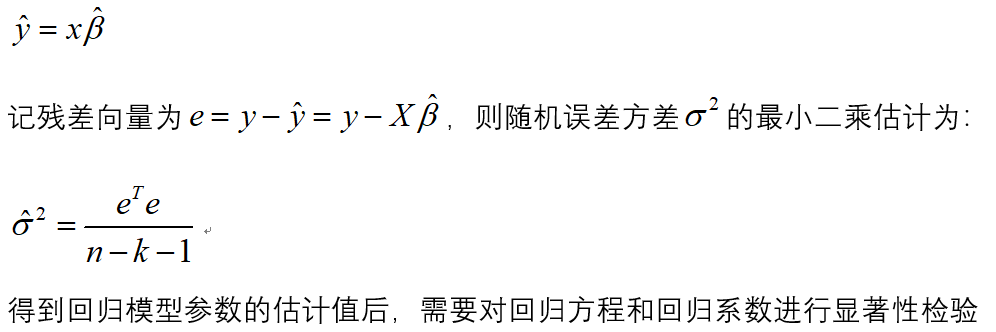（1）回归方程的显著性检验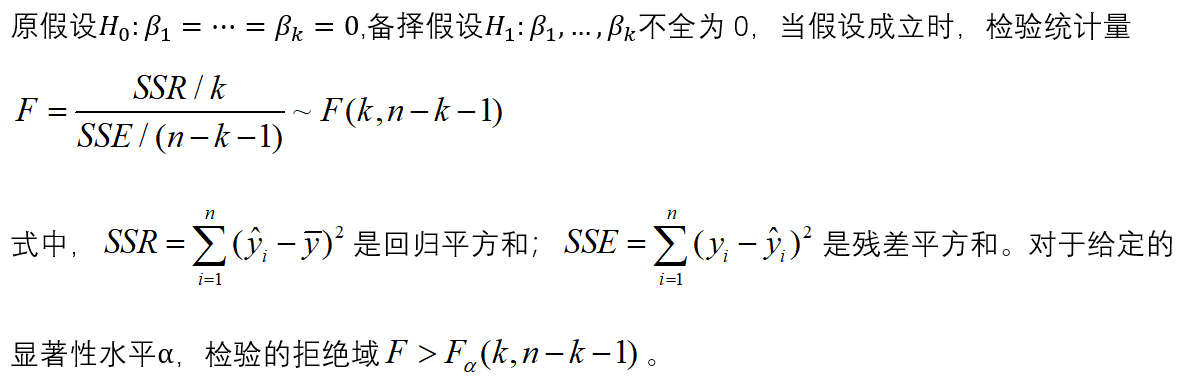（2）回归系数的显著性检验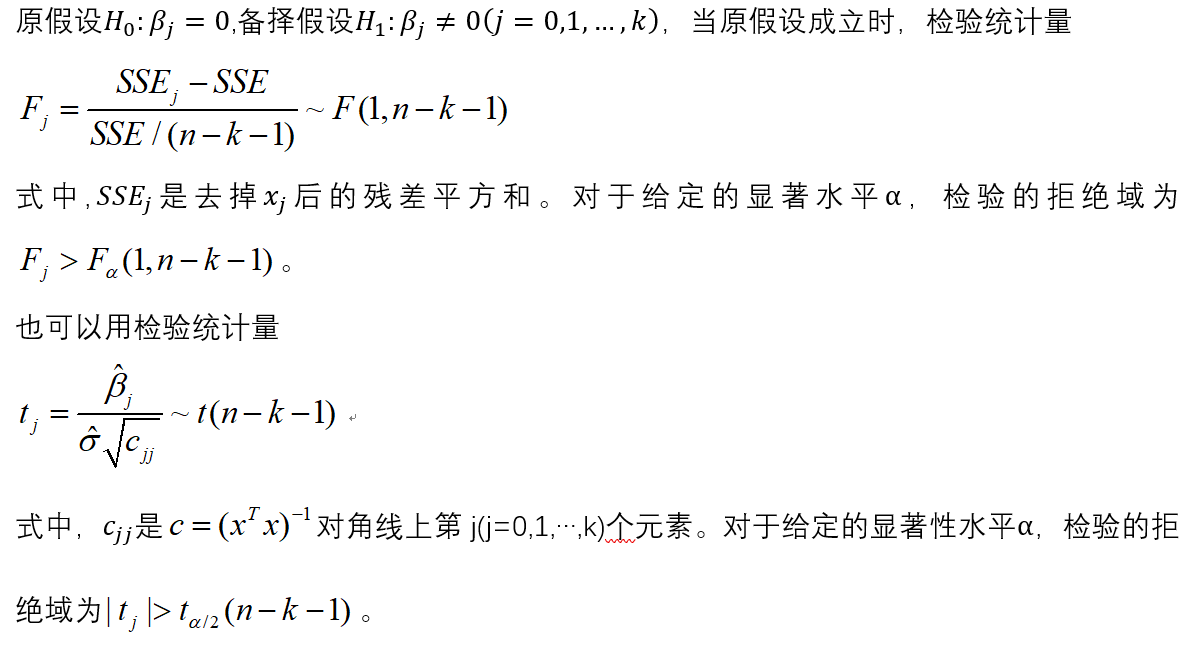2.建模步骤
（1）根据数据建立回归模型
（2）对模型进行显著性检验
（3)  对模型进行回归诊断
3.建模
library(car)
a
lm.salary=lm(锘縴~x1+x2+x3+x4,data=a)
summary(lm.salary)
#注：锘縴是y乱码之后的结果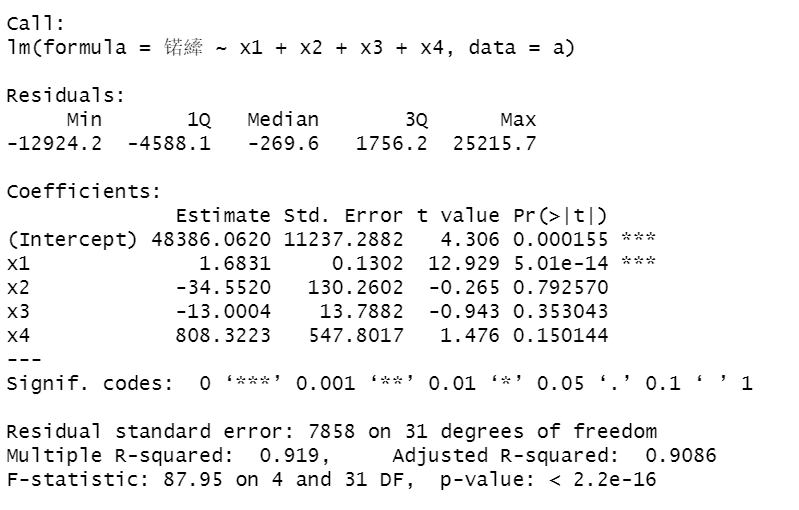发现x2,x3,x4系数不显著。
（2）对变量进行选择
lm.step=step(lm.salary,direction="both")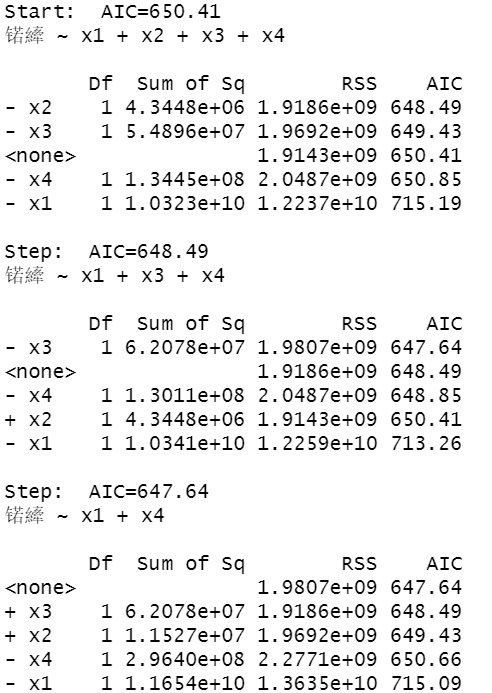如果去掉变量x2,AIC的值为648.49，如果去掉变量x3,AIC的值为650.85，如果去掉变量x1,AIC的值为715.19，所以在这里去掉x2.
进行下一轮的计算：
lm.salary=lm(锘縴~x1+x3+x4,data=a)
lm.step=step(lm.salary,direction="both")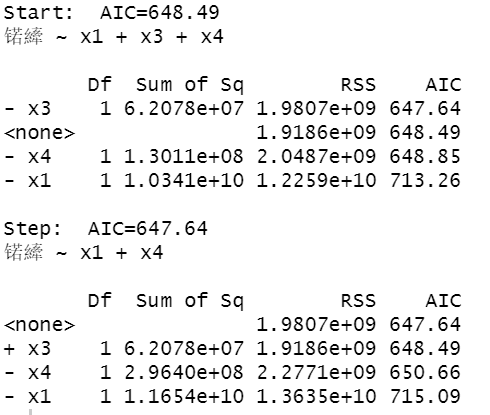发现去掉x3，AIC 的值为647.64，所以去掉x3.
单独对x1和x4，进行拟合。
lm.salary=lm(锘縴~x1+x4,data=a)
summary(lm.salary)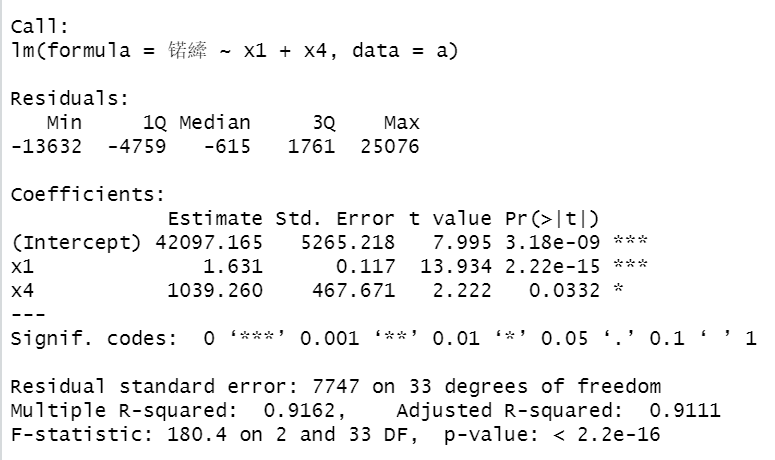可以看出F检验P值小于0.05显著，各个参数系数也是显著的。
（3）对上述回归模型进行回归残差诊断
算出模型的标准化残差
library(TSA)
y.rst=rstandard(lm.step)
y.rst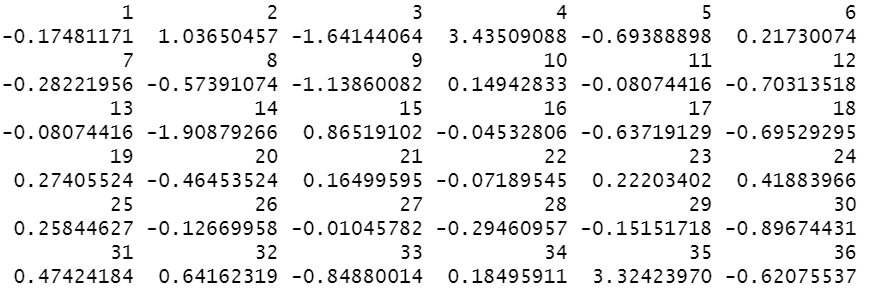画出其残差散点图：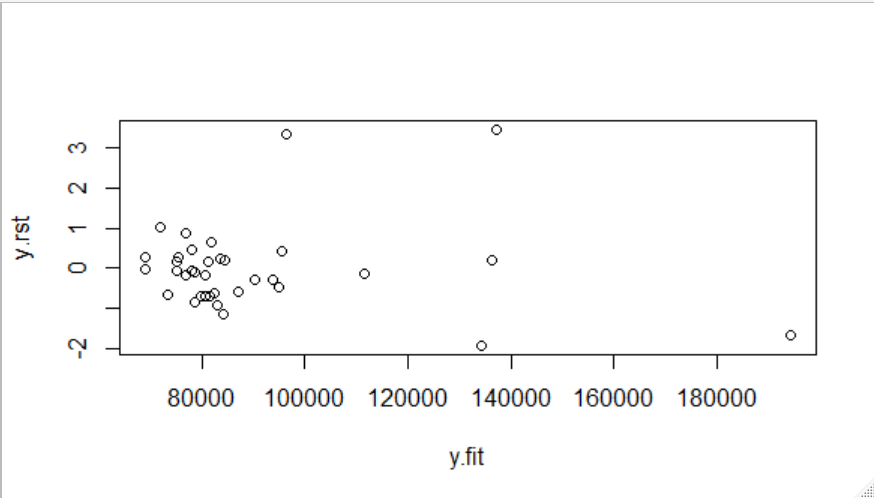很明显发现4和35号点异常，将这两个点去除。
lm.salary=lm(log(锘縴)~x1+x2+x3+x4,data=a[-c(4,35),])
lm.step=step(lm.salary,direction="both")
y.rst=rstandard(lm.step)
y.fit=predict(lm.step)
plot(y.rst~y.fit)

去除两点后的结果：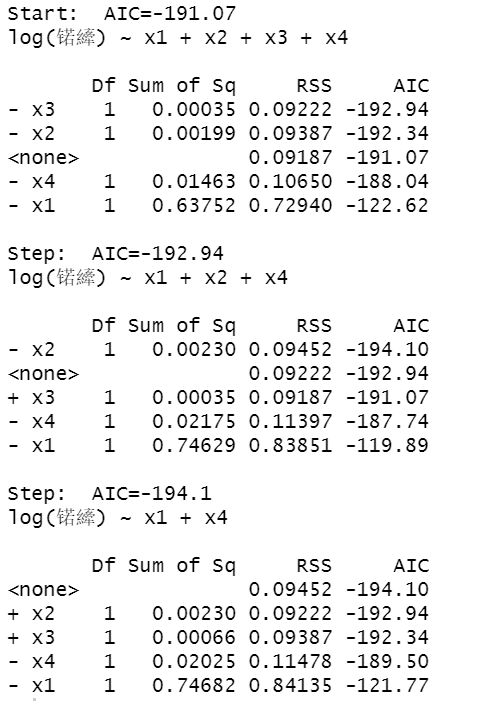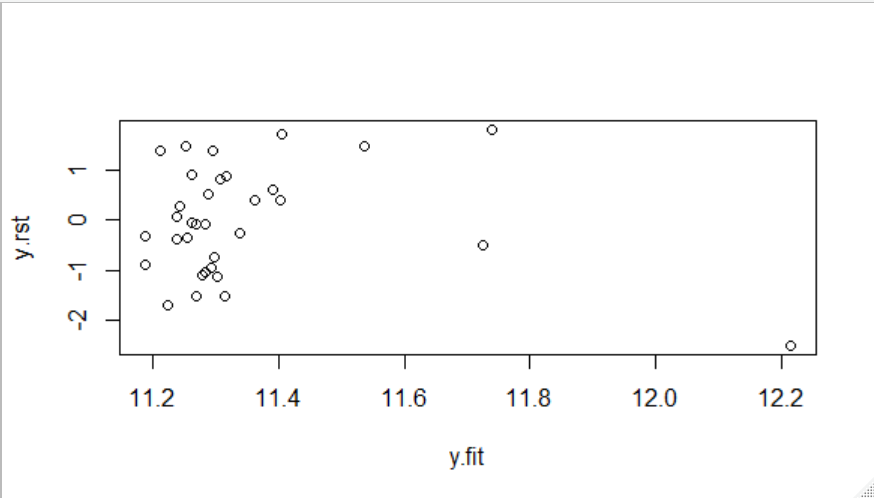绘制模型诊断图：
par(mfrow=c(2,2))
plot(lm.step)
influence.measures(lm.step)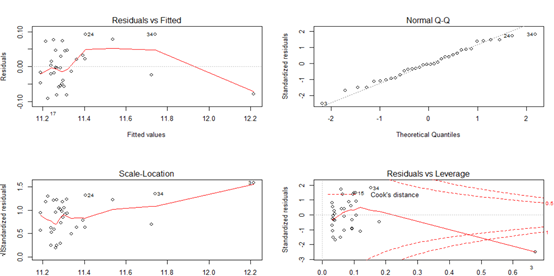残差拟合图基本上呈现随机分布模式，正态Q-Q图基本落在直线上，表明残差服从正态分布；大小-位置图和残差-杠杆图以小组的形式存在并且离中心不远。这说明3，4，35号观测值可能是异常点和强影响点。


展开全文• ## 多元相关分析与多元回归分析

万次阅读 多人点赞 2018-10-27 17:13:02
多元回归分析模型建立 线性回归模型基本假设 多元回归分析用途 多元线性相关分析 矩阵相关分析 复相关分析 曲线回归模型 多项式曲线 二次函数 对数函数 指数函数 幂函数 双曲线函数 变量间的...
目录

变量间的关系分析

什么是相关分析

什么是回归分析

分析步骤

回归分析与相关分析的主要区别

一元线性相关分析

一元线性回归分析

建模

方差分析检验

t检验

多元回归分析模型建立

线性回归模型基本假设

多元回归分析用途

多元线性相关分析

矩阵相关分析

复相关分析

曲线回归模型

多项式曲线

二次函数

对数函数

指数函数

幂函数

双曲线函数

变量间的关系分析

变量间的关系有两类，一类是变量间存在着完全确定的关系，称为函数关系，另一类是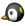变量间的关系不存在完全的确定性，不能用精缺的数学公式表示，但变量间存在十分密切的关系，这种称为相关关系，存在相关关系的变量称为相关变量。

相关变量间的关系有两种：一种是平行关系，即两个或两个以上变量相互影响。另一种是依存关系，即是一个变量的变化受到另一个或多个变量的影响。相关分析是研究呈平行关系的相关变量之间的关系。而回归分析是研究呈依存关系的相关变量间的关系。表示原因的变量称为自变量-independent variable，表示结果的变量称为因变量-dependent variable。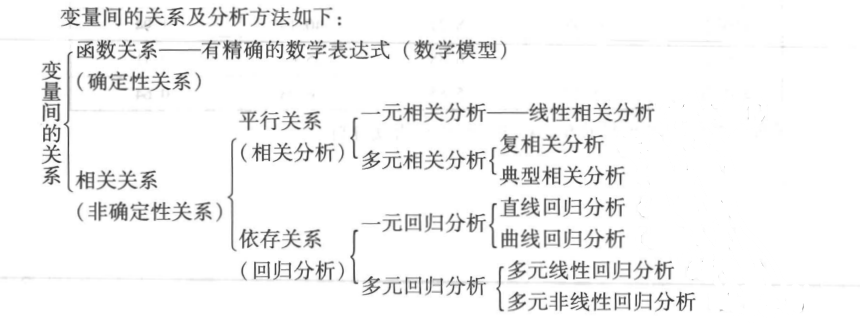什么是相关分析

通过计算变量间的相关系数来判断两个变量的相关程度及正负相关。

什么是回归分析

通过研究变量的依存关系，将变量分为因变量和自变量，并确定自变量和因变量的具体关系方程式

分析步骤

建立模型、求解参数、对模型进行检验

回归分析与相关分析的主要区别

1.在回归分析中，解释变量称为自变量，被解释变量称为因变量，相关分析中，并不区分自变量和因变量，各变量处于平的地位。--（自变量就是自己会变得变量，因变量是因为别人改变的）

2.在相关分析中所涉及的变量全部是随机变量，在回归分析中只有只有因变量是随机变量。

3.相关分析研究主要是为刻画两类变量间的线性相关的密切程度，而回归分析不仅可以揭示自变量对因变量的影响大小，还可以由回归方程进行预测和控制。

一元线性相关分析

线性相关分析是用相关系数来表示两个变量间相互的线性关系，总体相关系数的计算公式为：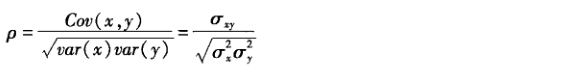δ^2x代表x的总体方差， δ^2y代表y的总体方差，δxy代表x变量与y变量的协方差，相关系数ρ没有单位，在-1到1之间波动，绝对值越接近1越相关，符号代表正相关或复相关。

一元线性回归分析

使用自变量与因变量绘制散点图，如果大致呈直线型，则可以拟合一条直线方程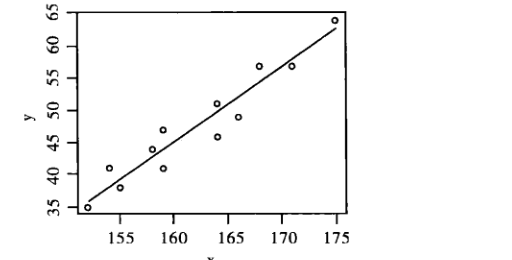建模

直线模型为：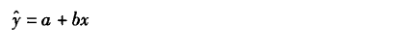y是因变量y的估计值，x为自变量的实际值，a、b为待估值

几何意义：a是直线方程的截距，b是回归系数

经济意义：a是x=0时y的估计值，b是回归系数

对于上图来说，x与y有直线的趋势，但并不是一一对应的，y与回归方程上的点的差距成为估计误差或残差，残差越小，方程愈加理想。

当误差的平方和最小时，即Q，a和b最合适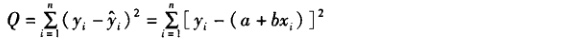对Q求关于a和b的偏导数，并令其分别等于零，可得：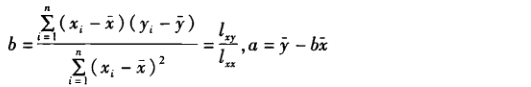式中，lxx表示x的离差平方和，lxy表示x与y的离差积和。

方差分析检验

将因变量y实测值的离均差平方和分成两部分即使：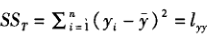分为：

实测值yi扣除了x对y的线性影响后剩下的变异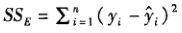和x对y的线性影响，简称为回归评方或回归贡献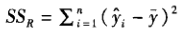然后证明：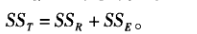t检验

当β成立时，样本回归系数b服从正态分布，这是可以使用T检验判断是否有数学意义，检验所用统计量为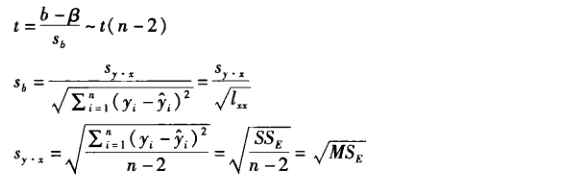例如t=10，那么可以判断α=0.05水平处拒绝H0，接受H1，那么x与y存在回归关系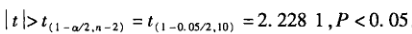多元回归分析模型建立

一个因变量与多个自变量间的线性数量关系可以用多元线性回归方程来表示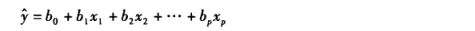b0是方程中的常数项，bi,i=1,2,3称为偏回归系数。

当我们得到N组观测数据时，模型可表示为：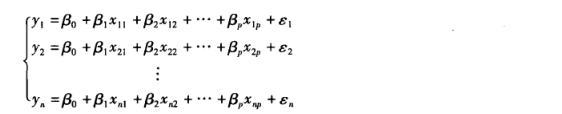其矩阵为：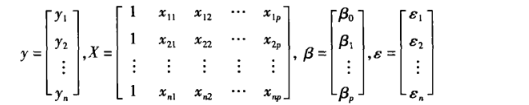X为设计阵，β为回归系数向量。

线性回归模型基本假设

在建立线性回归模型前，需要对模型做一些假定，经典线性回归模型的基本假设前提为：

1.解释变量一般来说是非随机变量

2.误差等方差及不相关假定（G-M条件）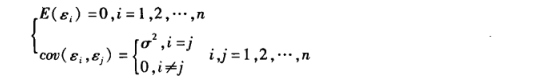3.误差正太分布的假定条件为：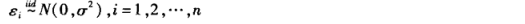4. n>p,即是要求样本容量个数多于解释变量的个数

多元回归分析用途

1.描述解释现象，希望回归方程中的自变量尽可能少一些

2.用于预测，希望预测的均方误差较小

3.用于控制，希望各个回归系数具有较小的方差和均方误差

变量太多，容易引起以下四个问题：
1.增加了模型的复杂度

2.计算量增大

3.估计和预测的精度下降

4.模型应用费用增加

多元线性相关分析

两个变量间的关系称为简单相关，多个变量称为偏相关或复相关

矩阵相关分析

设n个样本的资料矩阵为：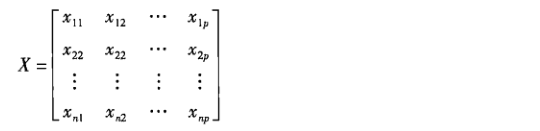此时任意两个变量间的相关系数构成的矩阵为：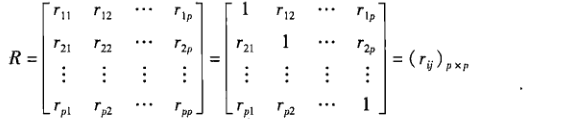其中rij为任意两个变量之间的简单相关系数，即是：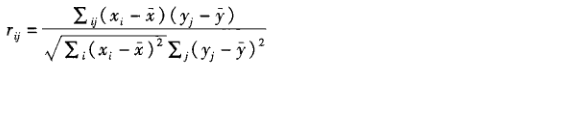复相关分析

系数计算：

设y与x1，x2，....，回归模型为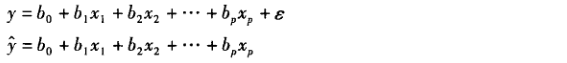y与x1，x2，....做相关分析就是对y于y^做相关分析，相关系数计算公式为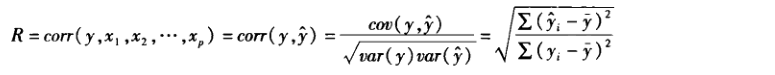曲线回归模型

多项式曲线

二次函数

y=a+bx+cx^2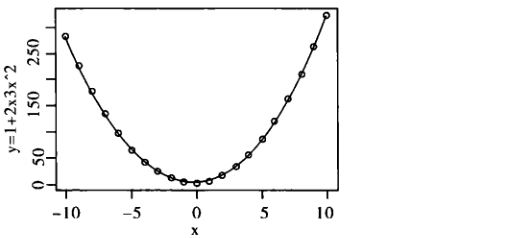对数函数

y=a+blogx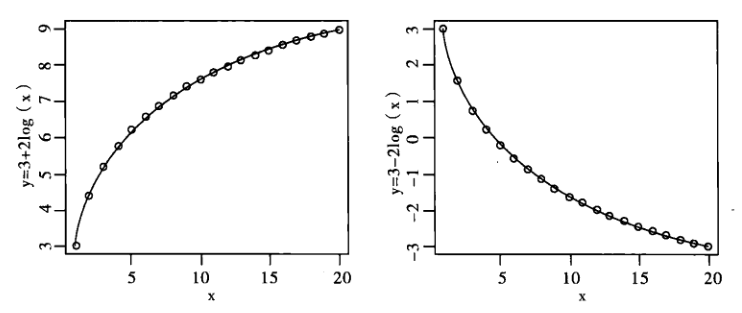指数函数

y = ae^bx或y = ae^(b/x)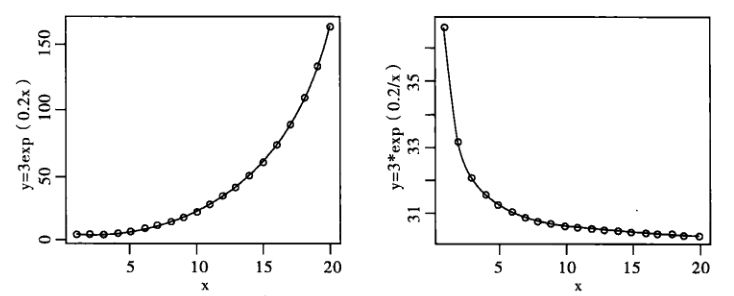幂函数

y=ax^b (a>0)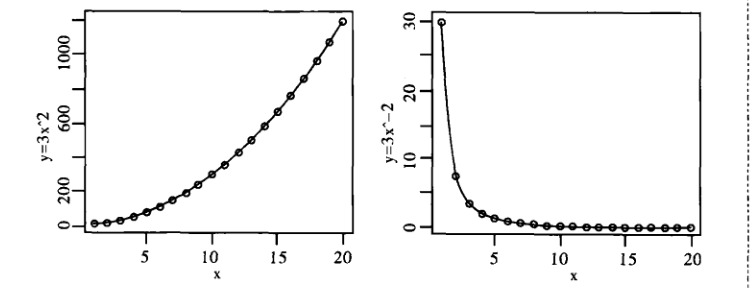双曲线函数

y = a+b/x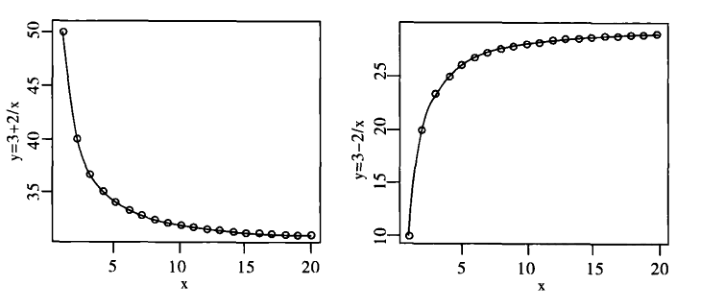实战操作见下一篇文章
展开全文• ## 多元回归

千次阅读 2019-04-13 21:02:58
多元回归 多元线性回归模型的基本假定 为了方便地进行模型的参数估计，我们对以下回归方程式有如下假定： Y^=Xβ+ε\hat{Y}=X\beta+\varepsilonY^=Xβ+ε 解释变量x1,x2,⋯&ThinSpace;,xpx_1,x_2,\cdots,x...
文章目录多元回归多元线性回归模型的基本假定参数估计量的性质回归方程的显著性检验中心化、标准化和相关系数与偏相关系数小结
多元回归
多元线性回归模型的基本假定
为了方便地进行模型的参数估计，我们对以下回归方程式有如下假定：
$\hat{Y}=X\beta+\varepsilon$

解释变量$x_1,x_2,\cdots,x_p$ 是确定性变量，不是随机变量，且要求$rank(X)=p+1<n$。这里的$rank(X)=p+1<n$，表明设计矩阵$X$中的自变量列之间不相关，样本量的个数应该大于解释变量的个数，$X$是以满秩矩阵。
随机误差项具有零均值等方差，即

$\begin{cases} E(\varepsilon_i)=0，\quad i =1,2,\cdots,n\\ cov(\varepsilon_i,\varepsilon_j)= \begin{cases} \sigma^2, &{i = j} \\ 0, & {i\neq j} \end{cases}\quad,i,j=1,2,\cdots,n \end{cases}$
这个假定常称为Gauss-Markov条件。$E(\varepsilon_i)=0$，即假设观察值没有系统误差，随机误差项$\varepsilon_i$的平均值为零。随机误差项$\varepsilon_i$的协方差为零，表明误差项在不同点之间是不相关的（在正态假定下即为独立的），不存在序列相关，并且有相同的精度。
参数估计量的性质
多元线性回归方程未知$\beta_0,\beta_1, ..., \beta_p$ 的估计是用最小二乘估计得到的。$\hat{y}=X\hat{\beta}=X(X^{'}X)^{-1}X^{'}y$中的$H=X(X^{'}X)^{-1}X^{'}$是一个帽子矩阵,可以证明其是$n$阶对称阵，同时还是幂等矩阵，即$H=H^2$。以下说一下参数估计量的性质：

$\hat{\beta}$是随机向量$y$的线性变换。
$\hat{\beta}$是$\beta$的无偏估计。
$D(\hat{\beta})=\sigma^2(X^{'}X)^{-1}$
高斯-马尔可夫定理：在假定的$E(y)=X\beta,D(y)=\sigma^2I_n$时，$\beta$的任一线性函数$c^{'}\beta$的最小方差线性无偏估计$(BLUE)$为$c^{'}\beta$，其中，$c$是任一$p+1$维常数向量，$\hat{\beta}$是${\beta}$的最小二乘估计。
读者在阅读高斯-马尔可夫定理的时候应该注意一下几点：可能存在非线性的，有偏估计使得方差不是最小。在正态假定下，即不可能存在$y_1,y_2,\cdots,y_n$非线性函数,，也不可能存在$y_1,y_2,\cdots,y_n$的其他非线性函数，作为$c^{'}\beta$的无偏估计，比最小二乘估计$c^{'}\hat{\beta}$的方差更小。
$cov(\hat{\beta},e)$=0
当$y = N(X\beta,\sigma^2I_n)$时，则

$\hat{\beta}$~$N(\beta,\sigma^2(X^{'}X)^{-1})$
$\frac{SSE}{\sigma^2}$~$X^2(n-p-1)$

回归方程的显著性检验
最小二乘法只是定性地给出了线性回归模型，但模型是否有效或者回归系数是否显著还需要经过检验才行。一般对线性回归模型进行两项统计检验：1、回归方程显著性$F$检验。2、回归系数显著性$t$检验。同时介绍衡量回归拟合程度的拟合优度检验。

$F$检验
$F$检验的原假设是:$H_0 :\beta_1=\beta_2,\cdots,\beta_p=0$,当$F>F_{\alpha}(p,n-p-1)$时，拒接原假设，认为在显著水平$\alpha$下,$y$与$x_1,x_2,\cdots,x_p$有显著关系，即方程回归显著的。也可以看它的$p$值，当$p<\alpha$时,拒绝原假设。

$t$检验
$t$检验的目是检验单个回归系数是否显著的，从而达到剔除多余无效变量的作用。注意由于各个变量之间是有交互作用的，因此不能一次剔除多个不显著变量，原则上每次只剔除一个变量，先剔除其中$t$值最小的（或者$p$值最大的）一个变量，然后再对求的得的新的回归回归方程进行检验，有不显著的变量再剔除，直到保留的变量对$y$有显著影响为止。在一元回归中，这两个检验是等价的，但是在多元检验中两者是不等价的。

拟合优度
拟合优度用于检验回归方程对样本观察值的拟合程度。样本决定系数：$R^2=\frac{SSR}{SST}=1-\frac{SSE}{SST}$
当样本量$n$和回归变量数目差接近的时候，$R^2$接近1，隐含虚假成分，因此在用这个决定系数决定模型的优劣一定要慎重。

中心化、标准化和相关系数与偏相关系数
在解释中心化之前先介绍一下舍入误差，及病态矩阵的概念。舍入误差主要是四舍五入等导致的误差，产生舍入误差产生的主要原因有二：①数据之间的量级悬殊；②设计矩阵$X$的列向量近似线性相关，导致$X^{'}X$为病态矩阵，其逆矩阵$(X^{'}X)^{-1}$就会产生较大的误差。病态矩阵：方程$AX=B$当其解集对$A$高度敏感，那么称$A$为病态矩阵。为了解决数据量纲和舍入误差的影响，因此要中心化和标准化。
1.中心化
中心化变换只改变直线截距，不改变直线斜率。通过坐标系的平移变换，使坐标原点移至样本中心，即做变换：
$\begin{cases}{x_j}^{'}=x_{ij}-\bar{x_j}\\{y_i}^{'}=y_i-\bar{y}\end{cases}$
2.标准化及标准化回归系数
为了消除量纲不同和数量级的差异带来的影响，就需要将数据标准化处理。让后利用得到标准化处理后的数据进行最小二乘估计位置参数，得到标准化回归系数。
3.复相关系数$R$反映的是$y$与一组自变量之间的相关关系，是整体和共性指标简单相关系数反映两个自变量间的相关性，是局部和个性指标。
4.偏相关系数可以度量$p+1$个变量$y,x_1,x_2,\cdots,x_p$之中任意两个变量的线性程度，而这种线性相关程度实在固定其余$p+1$个变量的影响下的线性相关。
小结
我们一般用最小二乘法进行模型的参数估计，不过在之前，我们要检验样本数据是否满足高斯-马尔可夫条件。初步得到模型以后，我们要做的是检验模型是否反映了线性关系，模型是否有效，模型参数是否显著。模型不通过可能有以下几方面的原因：①问题应该用非线性取描述，而错误地用线性进行拟合；由于认识的局限性，把重要的自变量给露了。用$R^2$进行的时候，要注意自变量个数要远小于样本量个数，这时的拟合优度检验才不会出现虚假因素。在存在交互现象的时候，用控制变量的方法解释单个参数对模型的影响是不合理的。


展开全文• 多元回归或复回归（multiple regression）:依变数依两个或两个以上自变数的回归。 主要内容： （1）确定各个自变数对依变数的单独效应和综合效应，建立由各个自变数描述和预测依变数反应量的多元回归方程。 （2）...
• 回归系数解释：回多元回归情形下，对每一个回归系数的解释如下，当所有其他自变量保持不变时，bi是因变量y对应于自变量xi改变一个单位时所做的改变的估计值。 多元判定系数（R-sq）：计算方法同简单线性回归，...统计学
• 前言：当影响因变量的因素是多个时候，这种一个变量同时与多个变量的回归问题就是多元回归，分为：多元线性回归和多元非线性回归。线性回归(Linear regressions)和逻辑回归(Logistic regressions)是人们学习算法的第...机器学习算法
• 最近做了一些用SPSS进行线性回归的实验，还是感觉很多细节把握不好，这里结合我的实验结果，以及网上别人的介绍总结一下，先贴几张SPSS的输出： 下面简单解释一下这三张图中的结果： 第一个表模型汇总表中，...
• 单变量线性回归 绘制散点图 相关系数R 拆分训练集和测试集 多变量线性回归 数据检验（判断是否可以做线性回归） 训练线性回归模型 先甩几个典型的线性回归的模型，帮助大家捡起那些年被忘记的数学。 ● 单...python 回归分析
• ## 多元线性回归推导过程

万次阅读 多人点赞 2018-08-06 20:26:23
常用算法一 多元线性回归详解1 此次我们来学习人工智能的第一个算法:多元线性回归.文章会包含必要的数学知识回顾,大部分比较简单,数学功底好的朋友只需要浏览标题,简单了解需要哪些数学知识即可. 本章主要包括...人工智能 最小二乘法
• 当影响因变量的因素是多个时候，这种一个变量同时与多个变量的回归问题就是多元回归，分为：多元线性回归和多元非线性回归。这里直说多元线性回归。对比一元线性回归： 1.1多元回归模型： y=β0+β1x1+β2x2+…+β...
• ## 多元线性回归 stata

千次阅读 多人点赞 2019-09-16 09:43:18
回归的思想，任务，使命 可以看到0-1回归的因变量自变量都是0-1变量 因变量y可以分为 解释y的形成机制就是通过建立x，y的数学关系式 感觉相关分析就是回归分析的前两步， 数据的分类 ...
• 现在用 Python 写线性回归的博客都快烂大街了，为什么还要用 SPSS 做线性回归呢？这就来说说 SPSS 存在的原因吧。 SPSS 是一个很强大的软件，不用编程，不用调参，点巴两下就出结果了，而且出来的大多是你想要的。...数据建模 Python
• 回归分析是数据分析中最基础也是最重要的分析工具，绝大多数的数据分析问题，都可以使用回归的思想来解决。回归分析的任务就是， 通过研究自变量X和因变量Y的相关关系，尝试去解释Y的形成机制，进而达到通过X去预测Y...异方差检验 多重共线性 逐步回归
• ## spss进行多元线性回归并分析表格

万次阅读 多人点赞 2019-04-01 00:50:26
1.如何使用spss进行多元线性回归。 2.分析生成结果，并判断回归是否可行。 一、使用spss进行多元线性回归： 1.输入数据 2. 3. 4. 5. 6. 二、表格结果分析： R方是检验回归是否成功的重要要素...
• ## 多元线性回归 及其Python实现

万次阅读 多人点赞 2019-04-05 21:14:11
多元线性回归 Python实现 多元线性回归求解过程 多元线性回归的形式： 目标函数： 将一个样本的向量化： 将所有样本的向量化： 向量化后的目标函数及求解结果： ps.上述多元线性回归的正规方程解问题是：事件...Python
• 接着上一次的一元线性回归...1、什么是多元线性回归模型？ 当y值的影响因素不唯一时,采用多元线性回归模型。 y =y=β0+β1x1+β2x2+...+βnxn 例如商品的销售额可能不电视广告投入,收音机广告python
• ## 多元线性回归的基础理解

万次阅读 多人点赞 2018-11-19 01:09:28
多元线性回归  Multivarate Linear Regression Multiple Feature 前面我们学习了一元线性回归，也动手亲自从底层编写了梯度下降算法来实现一元线性回归。相信大家已经对梯度下降和线性回归有了很清晰的理解了。 ...机器学习 梯度下降 正规方程 特征缩放
• 在回归分析中，如果有两个或两个以上的自变量，就称为多元回归。事实上，一种现象常常是与多个因素相联系的，由多个自变量的最优组合共同来预测或估计因变量，比只用一个自变量进行预测或估计更有效，更符合实际。 ...matlab 一元线性回归
• 实现任意多个变量以最小二乘法拟合成的新线性方程，通过变量带入线性方程可求得任意的拟合值。文件首行为行号、列号，其余行为数据行。
• 多元线性回归:在回归分析中，如果有两个或两个以上的自变量，就称为多元回归。事实上，一种现象常常是与多个因素相联系的，由多个自变量的最优组合共同来预测或估计因变量，比只用一个自变量进行预测或估计更有效，...测绘工程
• MATLAB多元线性回归: 基于MATLAB的多元非线性回归模型.pdf 多元线性回归建模以及MATLAB和SPSS求解.pdf MATLAB语言在多元线性回归中的应用.pdf
• 这是一个完美的任意元线性回归模型，使用c语言编写，采用初等变换实现，使用非常方便，只有2个函数，只要把要用到的函数拷到你的程序中即可。里面还附上逆阵的测试程序方便你验证，源文件使用.cpp或记事本打开
• 根据随机变量y及自变量x0,x1...xm-1的n组观测值做线性回归分析，简单实例，有注释，Java实现
• 主要用于数学建模（Matlab）的学习，下载下来换成你的数据就可以用了。
• 线性回归，前面用Python从底层一步一个脚印用两种方法实现了回归拟合。在这个高级语言层出不穷的年代，这样做显然不明智，所以我考虑用优秀的数据分析工具——R语言（不敢说最...
• ## 多元线性回归方程原理及其推导

万次阅读 多人点赞 2018-10-21 14:19:22
多元线性方程原理及推导 概念 1.在统计学中，线性回归方程是利用最小二乘函数对一个或多个自变量之间关系进行建模的一种回归分析。这种函数是一个或多个称为回归系数的模型参数的线性组合。只有一个自变量的情况...线性回归
• 本资源包含了收集到的”多元线性回归“算法的介绍文档、Java实现以及Excel多元线性回归分析功能的使用方法。并基于同一份数据，分别用来源于网络的2种Java代码、Excel进行了计算，并对3者的计算结果进行了比较，以...
• 1、多元线性回归模型 1.1多元回归模型与多元回归方程 设因变量为y，k个自变量分别为，描述因变量y如何依赖于自变量和误差项ε的方程称为多元回归模型。其一般形式可表示为： 式中，为模型的参数，ε为随机误差...
• 常用算法一 多元线性回归详解2(解析解求解多元线性回归)  上一篇讲到什么是多元线性回归以及多元线性回归的推导过程详解，本章我们一起来看如何求得最优解，就是我们得到了多元线性回归到损失函数就是最小二乘公式...最小二乘求解...